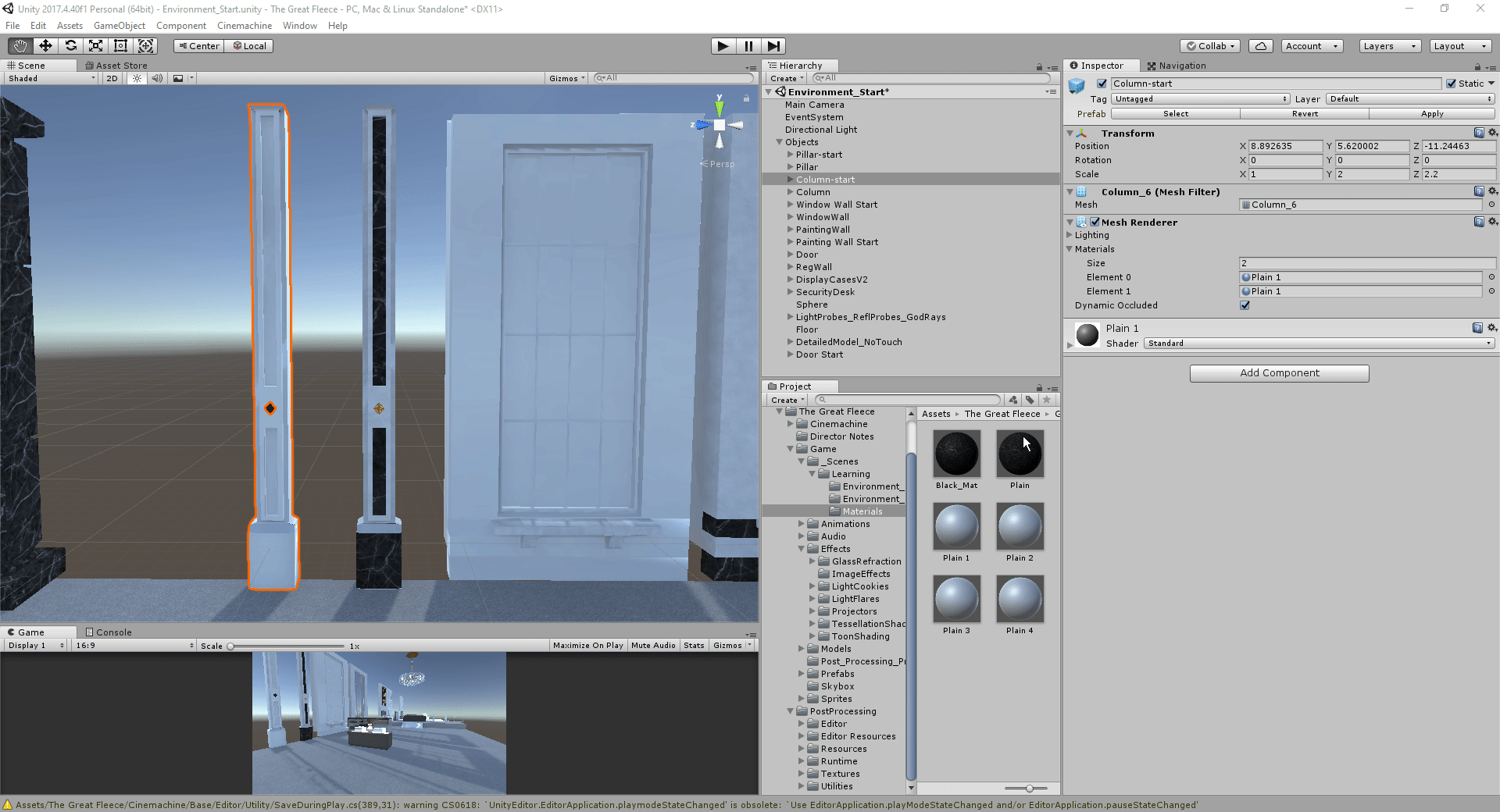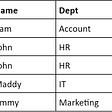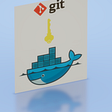# JOIN ON Condition in MaxCompute SQL

`A (LEFT/RIGHT/FULL/LEFT SEMI/LEFT ANTI) JOIN BON a.key = b.key and A.ds='20190121' and B.ds='20190121';`
`(SELECT * FROM A WHERE ds='20190121') A(LEFT/RIGHT/FULL/LEFT SEMI/LEFT ANTI)  JOIN(SELECT * FROM B WHERE ds='20190121') BON a.key = b.key`
1. WHERE condition of subquery
2. JOIN ON condition
3. WHERE condition after JOIN ON

# 1. Principle

`(SELECT * FROM A WHERE {subquery_where_condition} A) AJOIN(SELECT * FROM B WHERE {subquery_where_condition} B) BON {on_condition}WHERE {where_condition}`
1. {subquery_where_condition} in the subquery
2. {on_condition} of JOIN
3. The computing of the JOIN result set {where_condition}

# 2.1 Preparation

`CREATE TABLE A AS SELECT * FROM VALUES (1, 20190121),(2, 20190121),(2, 20190122) t (key, ds);`

# 2.2. Inner Join

`SELECT A.*, B.*FROM(SELECT * FROM A WHERE ds='20190121') AJOIN(SELECT * FROM B WHERE ds='20190121') BON a.key = b.key;`
`SELECT A.*, B.*FROM A JOIN BON a.key = b.key and A.ds='20190121' and B.ds='20190121';`
`SELECT A.*, B.*FROM A JOIN BON a.key = b.keyWHERE A.ds='20190121' and B.ds='20190121';`

# 2.3 Left Join

`SELECT A.*, B.*FROM(SELECT * FROM A WHERE ds='20190121') ALEFT JOIN(SELECT * FROM B WHERE ds='20190121') BON a.key = b.key;After filtering, there are two records on the left, one record on the right, and two records in the results.`
`SELECT A.*, B.*FROM A LEFT JOIN BON a.key = b.key and A.ds='20190121' and B.ds='20190121';`
`SELECT A.*, B.*FROM A LEFT JOIN BON a.key = b.keyWHERE A.ds='20190121' and B.ds='20190121';`

# 2.5 Full Join

`SELECT A.*, B.*FROM(SELECT * FROM A WHERE ds='20190121') AFULL JOIN(SELECT * FROM B WHERE ds='20190121') BON a.key = b.key;`
`SELECT A.*, B.*FROM A FULL JOIN BON a.key = b.key and A.ds='20190121' and B.ds='20190121';`
`SELECT A.*, B.*FROM A FULL JOIN BON a.key = b.keyWHERE A.ds='20190121' and B.ds='20190121';`

# 2.6 Left Semi Join

`SELECT A.*FROM(SELECT * FROM A WHERE ds='20190121') ALEFT SEMI JOIN(SELECT * FROM B WHERE ds='20190121') BON a.key = b.key;`
`SELECT A.*FROM A LEFT SEMI JOIN BON a.key = b.key and A.ds='20190121' and B.ds='20190121';`
`SELECT A.*FROM A LEFT SEMI JOIN(SELECT * FROM B WHERE ds='20190121') BON a.key = b.keyWHERE A.ds='20190121';`

# 2.7 Left Anti Join

`SELECT A.*FROM(SELECT * FROM A WHERE ds='20190121') ALEFT ANTI JOIN(SELECT * FROM B WHERE ds='20190121') BON a.key = b.key;`
`SELECT A.*FROM A LEFT ANTI JOIN BON a.key = b.key and A.ds='20190121' and B.ds='20190121';`
`SELECT A.*FROM A LEFT ANTI JOIN(SELECT * FROM B WHERE ds='20190121') BON a.key = b.keyWHERE A.ds='20190121';`

# 3. Summary

1. For INNER JOIN/LEFT SEMI JOIN, you can write filtering expressions anywhere.
2. For LEFT JOIN/LEFT ANTI JOIN, you need to put a filtering condition for the left table in {subquery_where_condition} or {where_condition} and a filtering condition for the right table in {subquery_where_condition} or {on_condition}.
3. RIGHT JOIN is opposite to LEFT JOIN: You need to put a filtering condition for the right table in {subquery_where_condition} or {where_condition} and a filtering condition for the left table in {subquery_where_condition} or {on_condition}.
4. For FULL OUTER JOIN, filtering conditions can only be put in {subquery_where_condition}.

--

--

--

## More from Alibaba Cloud

Follow me to keep abreast with the latest technology news, industry insights, and developer trends. Alibaba Cloud website:https://www.alibabacloud.com

Love podcasts or audiobooks? Learn on the go with our new app.

## How To: Azure Data Factory CI/CD with Azure DevOps pipelines — The YAML WAY!## <Metaverse Alliance: METASTRIKE>## MY REFLECTIONS ON ANDELA’S EPIC VALUES## Warm Disaster recovery for applications in Google Cloud## The Exciting World of 3D Elements: Albedo’s and Sub Meshes## The nteract March 2020 Release## Alibaba Cloud

Follow me to keep abreast with the latest technology news, industry insights, and developer trends. Alibaba Cloud website:https://www.alibabacloud.com

## Database Anomalies## Why Choose Cloud Data Storage Over On-premises Servers?## Row Oriented Database vs Column Oriented Database simplified.## Getting git to work in a Windows docker container